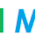### Problem

Given an array of strings, return all groups of strings that are anagrams.

An anagram is a type of word play, the result of rearranging the letters of a word or phrase to produce a new word or phrase, using all the original letters exactly once; for example Torchwood can be rearranged into Doctor Who.

#### Example :

"name", "mane" are anagrams

If two strings are anagram to each other, their sorted sequence is the same. Therefore, this problem can be seen as a problem of finding duplicate elements.

### JavaScript Code

```function anagrams(strs) {
var result = [];
if(strs == null || strs.length == 0)
return result;

var map = {};
for(var i=0; i<strs.length; i++){
var arr = strs[i].split('');
arr.sort();
var t = arr.join('');
if(map[t] == null){
var l = [];
l.push(i);
map[t] = l;
}else{
map[t].push(i);
}
}

for(var l in map){
if(map[l].length > 1){
for(var i=0; i<map[l].length; i++){
result.push(strs[map[l][i]]);
}
}
}

return result;
}

console.log(anagrams(['my','ym', 'name', 'mane', 'sriniv']));
```

#### 1 comment:

1.Words containing "Name"

There are Total 83 words containing this word. List of all words Containing Name are listed below categorized upon number of words.

Example : Jobnames, Surnamed, Enameler, Misnamed

Words Containing..

## BlogrollSrinivas Dasari find me on facebook follow me on twitter